085-1263404

### My Journal

All things Mathematical
30 Nov 2018
##### Question 1

A bullet of mass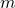is fired with speed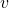into a fixed block of wood and is brought to rest in a distance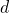. Find the resistance to motion assuming it to be constant.

Another bullet also of massis then fired with speed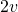into another fixed block of thickness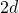, which offers the same resistance as the first block. Find the speed with which the bullet emerges, and the time it takes to pass through the block.

##### Question 2

Prove that the formula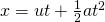represents the distance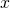travelled in timeby a body moving in a straight line with constant acceleration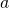.

A train takes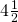minutes to travel between two stations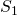and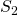which are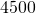meters apart. It starts from rest atand finishes at rest at, by travelling with uniform acceleration for the first minute and with uniform deceleration for the lastminute. Find the train’s constant speed during the remainder of the journey.

If a second train, travelling with a constant speed of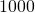m/min, in the same direction passesas the first train leaves this station, find when overtaking occurs. (The lengths of the trains may be neglected).

##### Question 3

A particle is projected under gravity with an initial velocity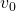at an angle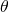to the horizontal. Find its position and the direction of motion after time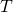in terms ofin terms of,,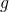and.

A particle is projected from the top of a cliff which is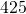ft. above sea level and the angle of projection is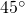to the horizontal. If the greatest height reached above the point of projection is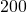ft, find the speed of projection and the time taken to reach this greatest height.

Find when and where the particles strikes the sea.

(Taketo be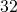ft/sec).

##### Question 4

Prove that the bob of a simple pendulum moves in simple harmonic motion – stating any assumptions made.

The string of such a pendulum is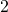ft. long and the bob is released from rest when at a distanceft from the equilibrium position. Calculate the time taken to travel halfway to the equilibrium position and the speed of the bob then.

(Taketo beft/sec).

##### Question 5

By deriving an expression for the necessary acceleration, prove that a particle of massmoving in a circle of radius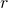with speedmust have a force of magnitude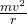pointing towards the centre acting on it.

A particle of mass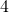lbs. moving on the inside smooth surface of a fixed spherical bowl of radiusft is describing a horizontal circle of radius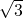ft. Find the constant speed of rotation and the reaction of the sphere on the particle.

(Taketo beft/sec).

##### Question 6

Show that the centre of gravity of a uniform triangular lamina coincides with the centre of gravity of three equal particles placed at the vertices of the triangle. Hence find the centre of gravity of a uniform trapezium, of weight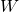, in which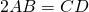and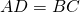.

A particle of weight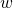is attached at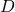and the system is suspended by a string attached to the midpoint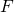of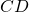. If in the position of equilibriumis vertically above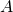show that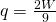.

##### Question 7

A particle of mass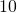lbs. is placed on a rough inclined plane. The least force acting up the plane which will prevent the particle slipping down the plane islbs. weight. The least force acting up the plane which will make the particle slip upwards islbs wt. Show that the coefficient of friction isand that the inclination of the plane is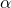where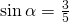.

Find the least force required to move the particle up the plane.

##### Question 8

Two equal uniform rods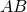and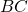each of weightare freely jointed at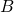. The system is suspended freely fromand a horizontal force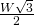is applied at the lowest point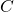. If in the equilibrium position the inclination ofto the downward vertical is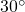, find the corresponding inclination ofand the supporting force at.

##### Question 9

A small uniform cylinder of density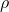, mass, total lengthand uniform cross section floats in a liquid of density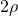with its axis vertical. Find the thrust on the cylinder when it is displaced vertically in the liquid, without being completely immersed, through a distancefrom the equilibrium position. Show that if it is released in this position, it will oscillate with simple harmonic motion of period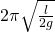.

##### Citation:

State Examinations Commission (2018). State Examination Commission. Accessed at: https://www.examinations.ie/?l=en&mc=au&sc=ru

Malone, D and Murray, H. (2016). Archive of Maths State Exams Papers. Accessed at: http://archive.maths.nuim.ie/staff/dmalone/StateExamPapers/

##### Licence:

“Contains Irish Public Sector Information licensed under a Creative Commons Attribution 4.0 International (CC BY 4.0) licence”.

The EU Directive 2003/98/EC on the re-use of public sector information, its amendment EU Directive 2013/37/EC, its transposed Irish Statutory Instruments S.I. No. 279/2005, S.I No. 103/2008, and S.I. No. 525/2015, and related Circulars issued by the Department of Finance (Circular 32/05), and Department of Public Expenditure and Reform (Circular 16/15 and Circular 12/16).

Note. Circular 12/2016: Licence for Re-Use of Public Sector Information adopts CC-BY as the standard PSI licence, and notes that the open standard licence identified in this Circular supersedes PSI General Licence No: 2005/08/01.